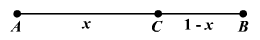# Golden Ratio

If a line segment is divided into two lengths such that the ratio of the segment’s entire length to the longer length is equal to the ratio of the longer length to the shorter length, then the segment has been divided into the Golden Ratio (also called the Golden Mean or Golden Proportion).

Take a line segment $\stackrel{¯}{AB}$ , then select a point $C$ on the segment such that $\frac{AC}{AB}=\frac{CB}{AC}$ .  If $AB=1$ , then $\frac{x}{1}=\frac{1-x}{x}$ .If you solve the resulting equation and calculate the appropriate ratio, it will yield the Golden Ratio $\frac{1+\sqrt{5}}{2}\approx 1.618033...$ .  It is often denoted by the Greek letter phi ( $\varphi$ ).

The Golden Ratio exists throughout creation. American researcher, Jay Hambridge, established that the Golden Ratio is the ratio of the height of a man to the length from his head to his navel.  Other researchers have claimed that ratios of other parts of the human body are also the Golden Ratio.  The lengths of the finger to the distance from the knuckle to the end of the finger, the length of the leg to the distance from the knee to the top of the leg and the distance from the shoulder to the fingertip to the distance from the elbow to the fingertip are all examples of the Golden Ratio.  It is also found in other natural objects such as nautilus seashells, and flowers whose leaves spiral around their stem.

The Golden Ratio appears often in architecture in places such as the ratio of the sides of the Great Temple, between the long and short sides of a pentagram   The ratio of the length to the height of the Parthenon in ancient Greece is also the Golden Ratio.

The length to width of the Golden Rectangle also equals the Golden Ratio.  It is thought by many to be the perfect rectangle and to be the most visually appealing of all geometric shapes.  It appears in many works of art and architecture.

The Fibonacci sequence is also related to the Golden Ratio.  If we form a sequence from the ratio of consecutive terms, $\frac{1}{1},\frac{2}{1},\frac{3}{2},\frac{5}{3},\frac{8}{5},\frac{13}{8},\frac{21}{13},...,\frac{144}{89},\frac{233}{144},...$ , the numbers approach the Golden Ratio.